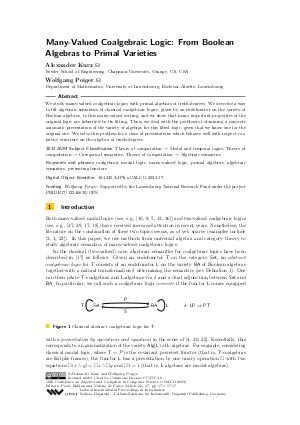Document# Many-Valued Coalgebraic Logic: From Boolean Algebras to Primal Varieties

### Authors Alexander Kurz, Wolfgang Poiger## File

LIPIcs.CALCO.2023.17.pdf
• Filesize: 0.7 MB
• 17 pages

## Cite As

Alexander Kurz and Wolfgang Poiger. Many-Valued Coalgebraic Logic: From Boolean Algebras to Primal Varieties. In 10th Conference on Algebra and Coalgebra in Computer Science (CALCO 2023). Leibniz International Proceedings in Informatics (LIPIcs), Volume 270, pp. 17:1-17:17, Schloss Dagstuhl - Leibniz-Zentrum für Informatik (2023)
https://doi.org/10.4230/LIPIcs.CALCO.2023.17

## Abstract

We study many-valued coalgebraic logics with primal algebras of truth-degrees. We describe a way to lift algebraic semantics of classical coalgebraic logics, given by an endofunctor on the variety of Boolean algebras, to this many-valued setting, and we show that many important properties of the original logic are inherited by its lifting. Then, we deal with the problem of obtaining a concrete axiomatic presentation of the variety of algebras for this lifted logic, given that we know one for the original one. We solve this problem for a class of presentations which behaves well with respect to a lattice structure on the algebra of truth-degrees.

## Subject Classification

##### ACM Subject Classification
• Theory of computation → Modal and temporal logics
• Theory of computation → Categorical semantics
• Theory of computation → Algebraic semantics
##### Keywords
• coalgebraic modal logic
• many-valued logic
• primal algebras
• algebraic semantics
• presenting functors

## Metrics

• Access Statistics
• Total Accesses (updated on a weekly basis)
0

## References

1. M. Bílková and M. Dostál. Expressivity of many-valued modal logics, coalgebraically. In J. Väänänen, Å. Hirvonen, and R. de Queiroz, editors, Logic, Language, Information, and Computation, pages 109-124. Springer Berlin Heidelberg, 2016.2. M. Bílková, A. Kurz, D. Petrişan, and J. Velebil. Relation lifting, with an application to the many-valued cover modality. Logical Methods in Computer Science, 9(4):739-790, 2013.3. P. Blackburn, M. de Rijke, and Y. Venema. Modal Logic. Cambridge Tracts in Theoretical Computer Science. Cambridge University Press, 2001.4. M. M. Bonsangue and A. Kurz. Presenting functors by operations and equations. In L. Aceto and A. Ingólfsdóttir, editors, Foundations of Software Science and Computation Structures, pages 172-186. Springer Berlin Heidelberg, 2006.5. F. Bou, F. Esteva, L. Godo, and R. O. Rodríguez. On the minimum many-valued modal logic over a finite residuated lattice. Journal of Logic and Computation, 21(5):739-790, 2011.6. S. Burris. Boolean powers. Algebra Universalis, 5:341-360, 1975.7. S. Burris and H.P. Sankappanavar. A Course in Universal Algebra. Graduate Texts in Mathematics. Springer, 1981.8. X. Caicedo and R. Rodriguez. Standard Gödel modal logics. Studia Logica, 94:189-214, 2010.9. C. Cîrstea and D. Pattinson. Modular construction of modal logics. In P. Gardner and N. Yoshida, editors, CONCUR 2004 - Concurrency Theory, pages 258-275. Springer Berlin Heidelberg, 2004.10. M. C. Fitting. Many-valued modal logics. Fundamenta Informaticae, 15(3-4):35-254, 1991.11. A. L. Foster. Generalized "Boolean" theory of universal algebras. Part i. Mathematische Zeitschrift, 58:306-336, 1953.12. G. Hansoul and B. Teheux. Extending Łukasiewicz logics with a modality: Algebraic approach to relational semantics. Studia Logica, 101:505-545, 2013.13. T.-K. Hu. Stone duality for primal algebra theory. Mathematische Zeitschrift, 110:180-198, 1969.14. T.-K. Hu. On the topological duality for primal algebra theory. Algebra Universalis, 1:152-154, 1971.15. B. Jacobs and A. Sokolova. Exemplaric expressivity of modal logics. Journal of Logic and Computation, 20(5):1041-1068, 2009.16. B. Klin. Coalgebraic modal logic beyond sets. Electronic Notes in Theoretical Computer Science, 173:177-201, 2007. Proceedings of the 23rd Conference on the Mathematical Foundations of Programming Semantics (MFPS XXIII).17. C. Kupke, A. Kurz, and D. Pattinson. Algebraic semantics for coalgebraic logics. Electronic Notes in Theoretical Computer Science, 106:219-241, 2004.18. C. Kupke and D. Pattinson. Coalgebraic semantics of modal logics: An overview. Theoretical Computer Science, 412(38):5070-5094, 2011.19. A. Kurz and R. Leal. Modalities in the Stone age: A comparison of coalgebraic logics. Theoretical Computer Science, 430:88-116, 2012. Mathematical Foundations of Programming Semantics (MFPS XXV).20. A. Kurz and D. Petrişan. Presenting functors on many-sorted varieties and applications. Information and Computation, 208(12):1421-1446, 2010.21. A. Kurz, W. Poiger, and B. Teheux. New perspectives on semi-primal varieties. Preprint available at https://arxiv.org/abs/2301.13406, 2023.
22. A. Kurz and J. Rosický. Strongly complete logics for coalgebras. Logical Methods in Computer Science, 8(3):1-32, 2012.23. C.-Y. Lin and C.-J. Liau. Many-valued coalgebraic modal logic: One-step completeness and finite model property. Preprint available at https://arxiv.org/abs/2012.05604, 2022.
24. M. Marti and G. Metcalfe. Expressivity in chain-based modal logics. Archive for Mathematical Logic, 57:361-380, 2018.25. Y. Maruyama. Algebraic study of lattice-valued logic and lattice-valued modal logic. In R. Ramanujam and S. Sarukkai, editors, Logic and Its Applications. ICLA, pages 170-184. Springer Berlin Heidelberg, 2009.26. Y. Maruyama. Natural duality, modality, and coalgebra. Journal of Pure and Applied Algebra, 216(3):565-580, 2012.27. L. S. Moss. Coalgebraic logic. Annals of Pure and Applied Logic, 96(1):277-317, 1999.28. E. Pacuit. Neighborhood Semantics for Modal Logic. Short Textbooks in Logic. Springer, 2017.29. D. Pattinson. Coalgebraic modal logic: Soundness, completeness and decidability of local consequence. Theoretical Computer Science, 309(1):177-193, 2003.30. D. Pattinson. Expressive logics for coalgebras via terminal sequence induction. Notre Dame Journal of Formal Logic, 45(1):19-33, 2004.31. R. W. Quackenbush. Primality: The influence of Boolean algebras in universal algebra. In Georg Grätzer. Universal Algebra. Second Edition, pages 401-416. Springer, New York, 1979.32. U. Rivieccio, A. Jung, and R. Jansana. Four-valued modal logic: Kripke semantics and duality. Journal of Logic and Computation, 27(1):155-199, 2017.33. A. Salibra, A. Bucciarelli, A. Ledda, and F. Paoli. Classical logic with n truth values as a symmetric many-valued logic. Foundations of Science, 28:115-142, 2023.34. A. Schiendorfer, A. Knapp, G. Anders, and W. Reif. MiniBrass: Soft constraints for MiniZinc. Constraints, 23(4):403-450, 2018.35. L. Schröder. Expressivity of coalgebraic modal logic: The limits and beyond. Theoretical Computer Science, 390(2):230-247, 2008. Foundations of Software Science and Computational Structures.36. A. Vidal, F. Esteva, and L. Godo. On modal extensions of product fuzzy logic. Journal of Logic and Computation, 27(1):299-336, 2017.X

Feedback for Dagstuhl Publishing